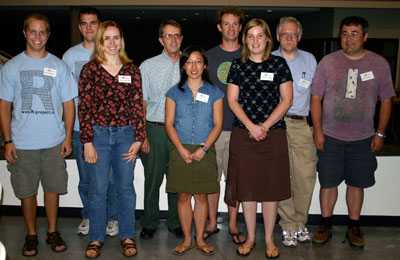## August 8–10. Iowa State University, Ames, Iowa

XLSolutions

ASA Sections on Statistical Graphics and Computing

Insightful

# Programming competition

## Winners

• First place equal: Elaine McVey, Olivia Lau
• Third place: Charlotte Wickham## Rules

You can use any resource on the web, apart from asking people questions (no asking on R help!)

Email your answers, as a single text file, to usercompetition@gmail.com. You are encouraged to submit answers as you complete them - and they may be revised with a final update at the end.

• 1 point for a solution
• 2 points for a clever solution
• 3 points for a solution that's cleverer than the model answer

Bonus points will be awarded for particularly elegant/concise/generalisable/well documented solutions.

Ties will be broken by submission time.

## Prizes

• Dinner with John Chambers at Hickory Park
• A book of your choice from Springer
• A book of your choice from CRC Press

### Relabelling observations

My client has recorded her observations as m1, m2, ..., m10, f1, ..., f10.

`obs <- c("f7", "f8", "m1", "m2", "m3", "f3", "m4", "f1", "m7", "m7", "f4", "m5", "f5", "m6", "f6", "m8", "m9", "f9", "m10", "f10", "f2")`

Actually, she should have recorded them as two variables. The first should be an integer variable corresponding to the integer portion of her observations, and the second should be a categorical variable with the two levels "male" and "female".

Your answer should be in the form of a function which takes the vector above and returns a data frame with two columns.

### Ragged longitudinal data

You have data with multiple observations per person and need to perform the following tasks

1. Find out how many people have 1,2,3,.. observations
2. Create a new variable that numbers the observations for each person as 1st, 2nd, 3rd,...
3. Given the name of a variable, create a new variable in each record showing the value that variable had at the previous measurement time.
4. Given the name of a variable that should be constant over time for an individual, check that it is actually constant.
5. Given a time point, find the last observation for each person before that time point and the first one after the time point (if any).

The data set has multiple observations per person, with people identified by values the 'id' variable.

`'visittime'` is the time that the observation was made. Everyone has an observation at time 0. `'futime'` is the end of follow-up for the person. Suitable variables for part 3 include '`chol`', '`ascites`', '`visittime`'. For part 4, suitable variables include '`trt`', '`agebl`','`sex`'.

### Folding functions

(a) Many binary operators in R have 'reducing' or 'folding' versions that collapse a vector to a single number

```"+"   sum()     1+2+3+4 == sum(c(1,2,3,4))
"*"   prod()    1*2*3*4 == prod(c(1,2,3,4))
"&"   all()     a & b & c == all(c(a, b, c))
"|"   any()     a | b | c == any(c(a, b, c))
```

Write a function `reduce(x, operator)` that generalizes this process to an arbitrary binary operator, so that `reduce(x,"+")` would be the same as `sum(x)`.

(b) The binary operators "+" and "*" have cumulative versions `cumsum()` and `cumprod()` so that

```cumsum(c(1,2,3,4)) = c(1, 1+2, 1+2+3, 1+2+3+4)
cumprod(c(1,2,3,4)) = c(1, 1*2, 1*2*3, 1*2*3*4)```

Write a function `accumulate(x, operator)` that generalizes this to an arbitrary binary operator, so that `accumulate(x, "+")` would be the same as `cumsum(x)`